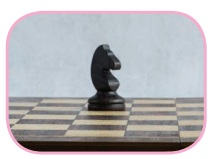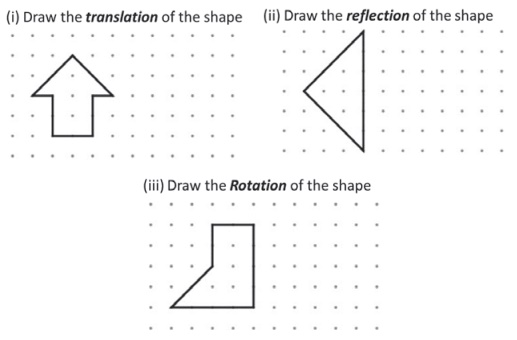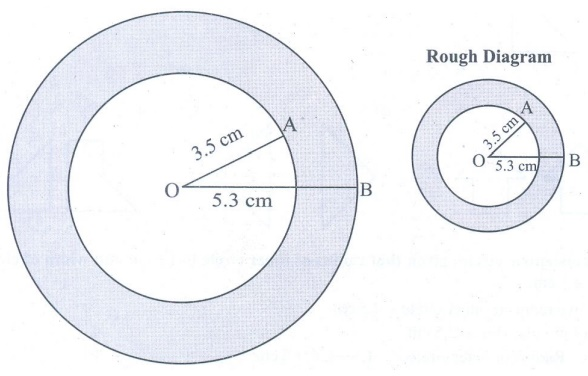Home | | Maths 7th Std | Exercise 4.3

# Exercise 4.3

7th Maths : Term 3 Unit 4 : Geometry : Miscellaneous Practice problems, Challenge Problems, Text Book Back Exercises Questions with Answers, Solution

Exercise 4.3

Miscellaneous Practice problems

1. The bishop, in given picture of chess board, can move diagonally along dark squares. Describe the translations of the bishop after two moves as shown in the figure.Solution:  For first move: 2→, 2↓; For second move: 5←, 5 ↓

2. Write a possible translation for each of chess piece for a single move.Solution:

Pawn – 1 ↑ or 2 ↑

Rook – 1 to 8 ↑

Knight – 2→, 1↑ or 2←, 1↑ or 1→, 2↑ or 1←, 2 ↑

Bishop – 1→, 1↑ or 2→, 2↑ or 3→, 3↑ or 4→, 4↑ or 5→ 5↑ 1 ←, 1↑ or 2 ←,2↑ or 3←,3↑ or 4←,4↑ or 5← 5↑

Queen – 1 to 8 ↑, 1→, 1↑ or 2→, 2 ↑or 3→, 3 ↑or 4→, 4↑or 5→, 5↑ or 1←, l↑ or 2← , 2↑ or 3←, 3 ↑or 4←, 4↑ or 5←5↑

King – 1 → or ←or ↑

3. Referring the graphic given, answer the following questions. Each bar of the category is made up of boy-girl-boy unit. (i) Which categories show a boy-girl-boy unit that is translation within the bar? (ii) Which categories show a boy-girl-boy unit that is reflected within the bar?Solution:

(i) Essay Writing category shows translation

(ii) Essay Writing and Mono Acting categories shows reflection

4. Given figure is a floor design in which the length of the small red equilateral triangle is 30 cm. All the triangles and hexagons are regular. Describe the translations in cm, represented by the (i) yellow line (ii) black line (iii) blue line.Solution:

(i) 120cm →, 210cm↓

(ii) 270 cm ←, 330cm↑

(iii) 150 cm →

5. Describe the transformation involved in the following pair of figures (letters). Write translation, reflection or rotation.Solution:

(i) rotation (ii) reflection (iii) translation (iv) reflection (v) rotation (vi) reflection (vii) rotation (viii) translation

Challenge Problems

6. In chess, a knight can move only in an L-shaped pattern:

● two vertical squares, then one horizontal square;

● two horizontal squares, then one vertical square;

● one vertical square, then two horizontal squares; or

● one horizontal square, then two vertical squares.

Write a series of translations to move the knight from g8 to g5 [at most two moves].Solution:  2←, 1↓ and then 1←, 2↓, (or) 2←, 1↓ and then 1 ←, 2 ↓

7. The pink shape is congruent to blue shape. Describe a sequence of transformations in which the blue shape is the image of pink shape.Solution:

(i) Translation 3←, 5↑ and 90° counter clockwise rotation about the green point and translates 5←, 2 ↓

(ii) Translation 2← 90° counter clockwise rotation about the green point and translates 2←, 2↓.

8.Solution:9. Draw concentric circles given that radius of inner circle is 4.5 cm and width of circular ring is 2.5 cm.

Solution:

Give radius of inner circle = 4.5 cm

Width of circular ring is 2.5 cm

Radius of outer circle = 4.5 + 2.5 = 7 cmStep 1 : Drawn a rough diagram and marked the given measurements

Step 2 : Taken any point O and marked it as the center.

Step 3 : With O as center and drawn a circle of radius OA = 4.5 cm.

Step 4 : With O as center drawn a circle of radius OB = 4.5 + 2.5 = 7 cm. Thus the concentric circles C1 and C2 are drawn.

Width of the circular ring = OB – OA

= 7 – 4.5 = 2.5 cm

10. Draw concentric circles given that radius of outer circle is 5.3 cm and width of circular ring is 1.8 cm.

Solution:

Give radius of outer circle = 5.3 cm

Width of circular ring = 1.8 cm

Radius of the inner circle = 5.3 – 1.8

= 3. 5cmStep 1 : Drawn a rough diagram and marked the given measurements

Step 2 : Taken any point O and marked it as the center.

Step 3 : With O as center drawn a circle of radius OA = 3.5 cm.

Step 4 : With O as center, drawn a circle of radius OB = 5.3 cm. Thus concentric circles C1 and C2 are drawn. Width of the circular ring = OB – OA

= 5.3 – 3.5 = 1.8 cm

Exercise 4.3

Miscellaneous Problems

1. For first move: 2→,2↓; For second move: 5←,5↓

2. Pawn – 1↑ or 2↑

Rook – 1 to 8 ↑

Knight – 2→,1↑ or 2←,1↑ or1→,2↑ or 1←,2↑

Bishop – 1→,1↑ or 2→,2↑or 3→,3↑or 4→,4↑or 5→5↑1←,1↑ or 2←,2↑ or 3←,3↑ or 4←,4↑ or 5←5↑

Queen – 1 to 8 ↑, 1→, 1↑ or 2→,2↑ or 3→,3↑ or 4→,4↑ or 5→,5↑ or 1←,1↑ or 2←,2↑ or 3←,3↑ or 4←,4↑ or 5←5↑

King – 1→ or ← or ↑

3. (i) More brains category shows translation

(ii) More brains category shows reflection

4. (i) 120cm→,210cm↓ (ii) 270cm←,330cm↑ (iii) 150cm

5. (i) rotation (ii) reflection (iii) translation (iv) reflection (v) rotation (vi) reflection (vii) rotation. (viii) translation

challenge Problems

6. 2←,1↓ and then 1←, 2↓ (or) 2←, 1↓ and then 1←,2↓

7. (i) Translation 3←,5↑ and 90° counter clockwise rotation about the green point and translates 5 ←, 2↓

(ii) Translation 2←90° counter clockwise rotation about the green point and translates 2←,2↓

8.Tags : Questions with Answers, Solution | Geometry | Term 3 Chapter 4 | 7th Maths , 7th Maths : Term 3 Unit 4 : Geometry
Study Material, Lecturing Notes, Assignment, Reference, Wiki description explanation, brief detail
7th Maths : Term 3 Unit 4 : Geometry : Exercise 4.3 | Questions with Answers, Solution | Geometry | Term 3 Chapter 4 | 7th Maths